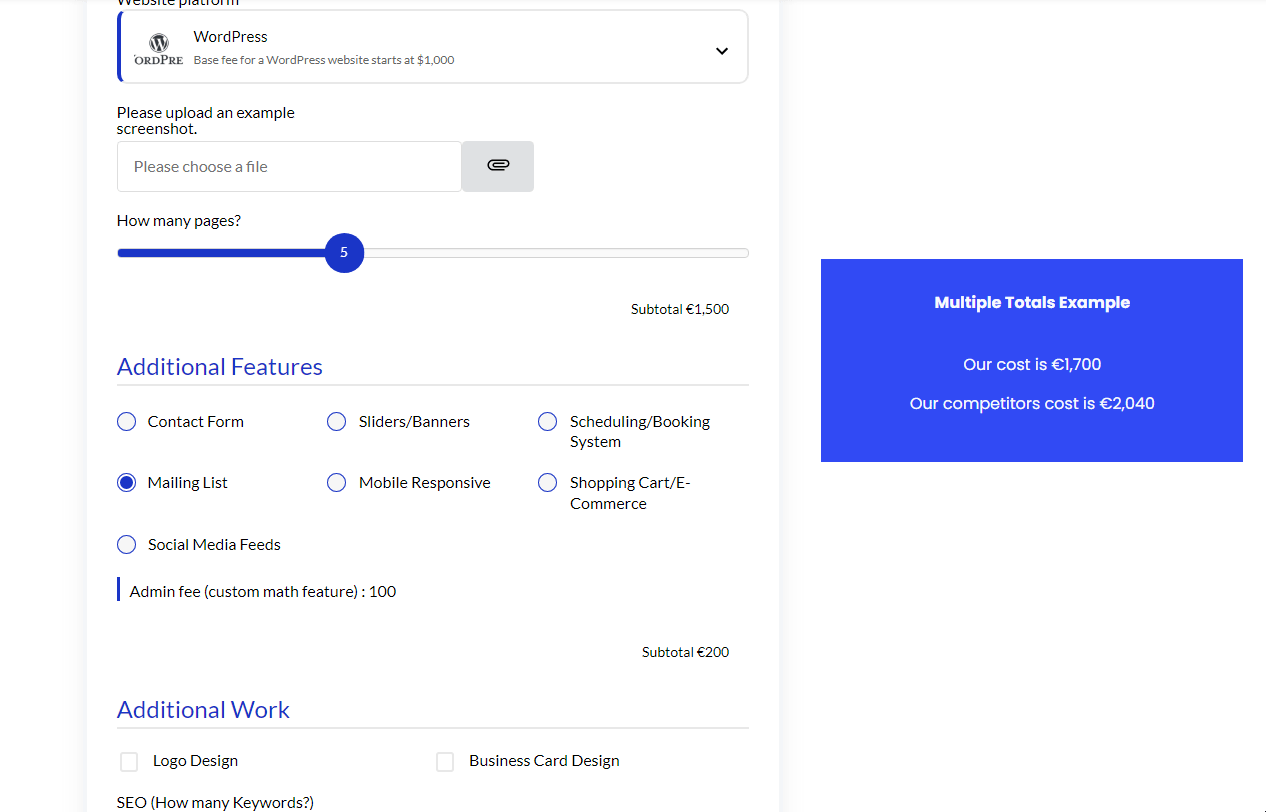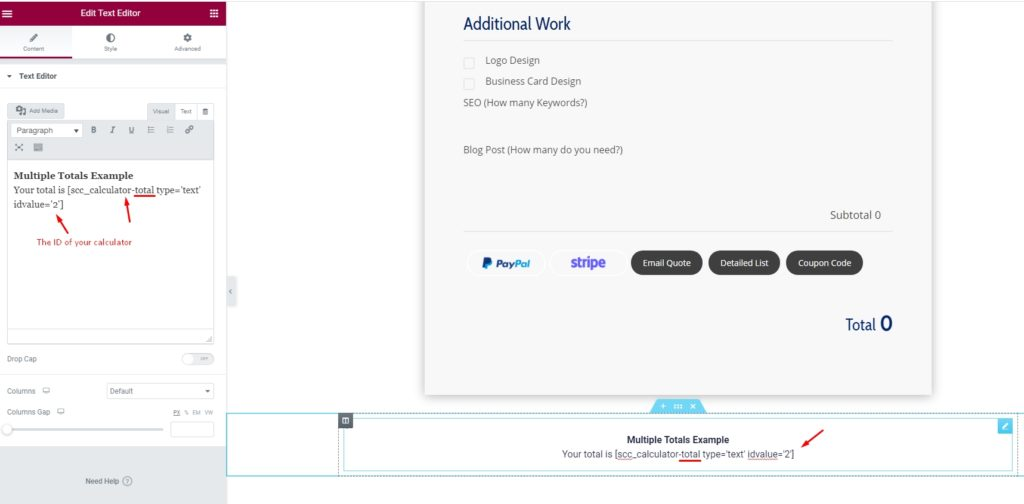Introduction

Show multiple results and custom totals by placing the calculator total anywhere on your website using a shortcode

You can either show the original total price or manipulate it with math for certain use cases (comparing competitive pricing and much more).Benefits, Features & Use Cases

Show multiple results by:

• Display the calculator's total cost again somewhere else on the page. Use CSS code to style the way you want.
• Combine two calculator totals that are on the same page.
• Competitor Pricing -  compare your pricing to a competitor's pricing. A custom total shortcode should be added and multiplied by 1.15 if their prices are 15% higher than yours.
• Show Monthly Pricing -  calculate the monthly cost by dividing the total price by 12
• Display a Price Range

Watch It In-Action

In this GIF example, we are showing what it would look like if you were to compare your competitor's pricing.How to Activate & Use This Feature

Settings & Options

Enter the IDs of each calculator as we did below. In this example, we're combining calculators #5 and #7

• Display the calculator total cost again somewhere else on the page
[scc_calculator-total type='text' idvalue='2']

Combine Multiple Calculators

Combine two calculator totals that are on the same page Enter the IDs of each calculator as we did below. In this example, we're combining calculators #5 and #7

[scc_calculator-total combine='5,7']

Currency Symbols

Use the currency-symbol attribute to disable the currency symbol.
This attribute works if you use the 'combine' attribute.

[scc_calculator-total combine='5,7' currency-symbol=0]

Currency Comma Format

Use the comma-separator attribute to switch the mode of the comma separator between 'default' and 'comma'. By default, the browser locale-based formatting will be applied. If you want to have the commas regardless of the region, set the parameter to comma-separator=comma

[scc_calculator-total combine='5,7' currency-symbol=0 comma-separator=comma]

Apply Math

To apply math operations over the total value or the combined total value, you can use the 'apply-math' attribute.

[scc_calculator-total combine='5,7' currency-symbol=0 apply-math="divide:2"]

Here, the 'divide:2' is the math type, followed by the value the total amount should be divided against. You can use the multiply, add, and subtract operation over the value returned by the shortcode. Here are the example.

[scc_calculator-total idvalue='2' apply-math="divide:2"]
(divides the value by 2)

[scc_calculator-total idvalue='2'  apply-math="multiply:20"]
(multiplies the value by 20)

[scc_calculator-total combine='5,7' currency-symbol=0 apply-math="subtract:2"]

(combines two calculators & subtracts 23 from the value)

In this example, we use Elementor to add the shortcode.Related Blog Post

Related Features

Limitations

• The custom totals can only show on your webpage, it cannot show on Email Quote, PDF or Detailed List View.